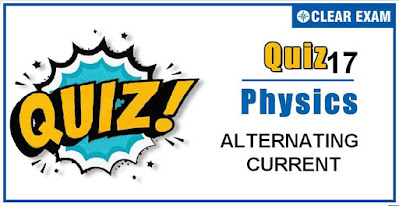## [LATEST]\$type=sticky\$show=home\$rm=0\$va=0\$count=4\$va=0

As per analysis for previous years, it has been observed that students preparing for NEET find Physics out of all the sections to be complex to handle and the majority of them are not able to comprehend the reason behind it. This problem arises especially because these aspirants appearing for the examination are more inclined to have a keen interest in Biology due to their medical background. Furthermore, sections such as Physics are dominantly based on theories, laws, numerical in comparison to a section of Biology which is more of fact-based, life sciences, and includes substantial explanations. By using the table given below, you easily and directly access to the topics and respective links of MCQs. Moreover, to make learning smooth and efficient, all the questions come with their supportive solutions to make utilization of time even more productive. Students will be covered for all their studies as the topics are available from basics to even the most advanced. .

Q1. A coil of resistance R and inductance L is connected to a battery of emf E volt. The final current in the coil is
•  E/R
•  E/L
•  √( (E/(R2+L2)) )
•  √( (EL/(R2+L2)) )
Solution
Final current in constant and L plays no role at that instant. Therefore, i=E/R.

Q2. An alternating e.m.f. is applied to purely capacitive circuit. The phase relation between e.m.f. and current flowing in the circuit is or In a circuit containing capacitance only
•  e.m.f. is ahead of current by π/2
•  Current is ahead of e.m.f. by π/2
•  Current lags behind e.m.f. by π
•  Current is ahead of e.m.f. by π
Solution
For purely capacitive circuit e=e0 sin⁡ωt i=i0 sin⁡(ωt+π/2),i.e., current is ahead of emf by π/2

Q3. What is the value of inductance L for which the current is a maximum in a series L-C-R circuit with C=10μFand ω=1000 s^(-1)?
•  100 mH
•  1 mH
•  Cannot be calculated unless R is known
•  10 mH
Solution
Current in a L-C-R series circuit,

Where V is rms value of current, R is resistance, XL is inductive reactance and XC is capacitive reactance.
For current to be maximum, denominator should be minimum which can be done, if
XL=XC
This happens in resonance state of the circuit
i.e, ωL=1/ωC
or L=1/(ω2C) ...(i)
Given, ω=1000s-1,C=10μF=10×10-6
Hence, L=1/(10002×10×10-6)=0.1 H=100 mH

Q4. What will be the self inductance of a coil, to be connected in a series with a resistance of π√3 Ω such that the phase difference between the emf and the current at 50 Hz frequency is 30°
•  0.5 henry
•  0.03 henry
•  0.05 henry
•  0.01 henry
Q5. When an AC source of emf e=E0 sin⁡(100t) is connected across a circuit, the phase difference between the emfe and the current i in the circuit is observed to beπ/4 , as shown in the diagram. If the circuit consists possibly only of R – C or R – L or L – C in series, find the relationship between the two elements
•  R=1 kΩ,C=10 μF
•  R=1 kΩ,C=1 μF
•  R=1 kΩ,L=10 H
•  R=1 kΩ,L=1 H
Solution
As the current i leads the emf e by π/4, it is an R – C circuit
tan ⁡ϕ =XC/R
or tan⁡ π/4 =(1/ωC)/R
The product of C – R should be 1/100 s-1.

Q6. The current in series LCR circuit will be maximum when ω is
•  As large as possible
•  Equal o natural frequency of LCR system
• √LC
•  √(1/LC)
Solution
At resonant frequency current in series LCR circuit is maximum

Q7. Three identical ring move with the same speed on a horizontal surface in a uniform horizontal magnetic field normal to the planes of the rings. The first (A) slips without rolling, the second (B) rolls without slipping, and the third rolls with slipping
•  The same emf is induced in all the three rings
•  No emf is induced in any of the rings
•  In each ring, all points are at the same potential
•  B developes the maximum induced emf, and A the least.
Solution
The same emf is induced in all the three rings because emf is only due to linear motion and does not depend on spin.

Q8. An alternating voltage e=200 sin⁡ 100 t is applied to a series combination R=30 Ω and an inductor of 400 mH. The power factor of the circuit is
•  0.01
•  0.2
•  0.05
•  0.6
Q9. An alternating e.m.f. of angular frequency ω is applied across an inductance. The instantaneous power developed in the circuit has an angular frequency
•  ω/4
•  ω/2
•  ω
•
Solution
The instantaneous values of emf and current in inductive circuit are given by E=E0 sin⁡ ωt and i=i0 sin⁡(ωt-π/2) respectively
So, Pinst=Ei=E0 sin ⁡ωt × i0 sin⁡(ωt-π/2)
=E0 i0sin⁡ ωt(sin ⁡ωt cos⁡ π/2-cos⁡ ωt sin⁡π/2)
=E0 i0sin⁡ωt cos⁡ωt
=1/2 E0 i0 sin⁡ 2ωt(sin ⁡2ωt = 2sin⁡ ωt cos⁡ ωt)
Hence, angular frequency of instantaneous power is 2ω

Q10. The peak value of an alternating emf E given by E=E0 cos⁡ωt is 10 V and its frequency is 50 Hz. At a time t=1/100 s, the instantaneous value of the emf is
•  10 V
•  5√3 V
•  5 V
• 1 V
Solution
E=E0 cos ⁡ωt= 10 cos⁡ (2π × ft) =10 cos⁡(2π × 50 × 1/600) =10 cos⁡(π/6)=10 × √3/2 = 5√3 V## Want to know more

Please fill in the details below:

## Latest NEET Articles\$type=three\$c=3\$author=hide\$comment=hide\$rm=hide\$date=hide\$snippet=hide

Name

ltr
item
BEST NEET COACHING CENTER | BEST IIT JEE COACHING INSTITUTE | BEST NEET, IIT JEE COACHING INSTITUTE: Quiz-17 ALTERNATING CURRENT
Quiz-17 ALTERNATING CURRENT